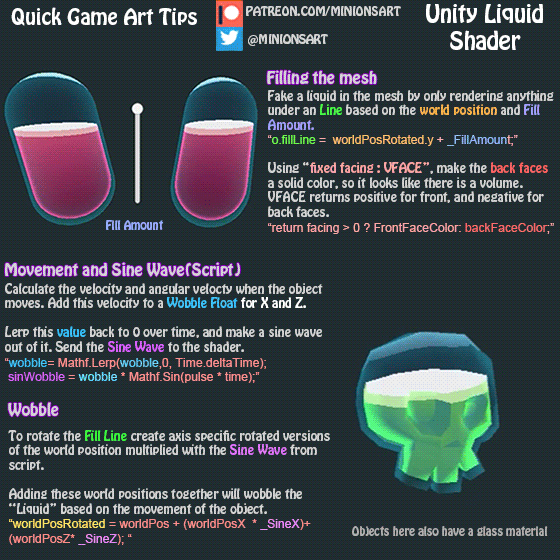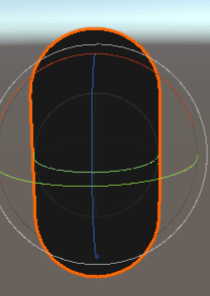# Moving/spilling water inside a cup of glass

I made a glass of water but there are somethings i didnt know how to do well, what i did is i put a mesh on the top of the glass to represent the surface of the water but it doesnt really work well with glass because if you look from the sides you wont really see that there is water in there, this is what i did :the glass from the side :as you can see it doesnt really work well in my case. I also tried putting an object that has the same shape as the cup inside to represent water but that would make it difficult for what i want to do next, because my next step would be to make the water rotate in the opposite rotation of the cup and make it spill if it reaches the top of the cup, and that is my second question how do i make the water spill from the cup?

Any help is very much appreciated.

• @SeyedMortezaKamali i'm very beginner when it comes to shaders been trying to avoid because they seem so hard but i'll eventually ned to learn them ^^, i kind of understand how a stencil buffer works but can you give me just some more info or sources that will help me to creat the shader neede? Sep 23, 2018 at 18:13

you can use world space to make this effect:the transformation from object space to world space is put into a 4×4 matrix, which is also known as “model matrix” (since this transformation is also known as “model transformation”). This matrix is available in the uniform parameter unity_ObjectToWorld, which is automatically defined by Unity in this way:

 uniform float4x4 unity_ObjectToWorld;


Since it is automatically defined, we don't need to define it (actually we must not). Instead we can use the uniform parameter unity_ObjectToWorld without definition in the following shader: https://en.wikibooks.org/wiki/Cg_Programming/Unity/Shading_in_World_Space

Shader "Smkgames/WaterFill" {
Properties{
_Fill("Fill",Range(-1,1)) = 0
_Pos("Pos",Vector) = (0,0,0,0)
}
Pass {
CGPROGRAM

#pragma vertex vert
#pragma fragment frag

// uniform float4x4 unity_ObjectToWorld;
// automatic definition of a Unity-specific uniform parameter

struct vertexInput {
float4 vertex : POSITION;
};
struct vertexOutput {
float4 pos : SV_POSITION;
float4 position_in_world_space : TEXCOORD0;
};
float _Fill;
float4 _Pos;
vertexOutput vert(vertexInput input)
{
vertexOutput output;

output.pos =  UnityObjectToClipPos(input.vertex);
output.position_in_world_space =
mul(unity_ObjectToWorld, input.vertex)-_Pos;
// transformation of input.vertex from object
// coordinates to world coordinates;
return output;
}

float4 frag(vertexOutput input) : COLOR
{
//  float dist = distance(input.position_in_world_space,
//    float4(0.0, 0.0, 0.0, 1.0));
// computes the distance between the fragment position
// and the origin (the 4th coordinate should always be
// 1 for points).

if (input.position_in_world_space.y < _Fill)
{
return float4(0.0, 1.0, 0.0, 1.0);
// color near origin
}
else
{
return float4(0.1, 0.1, 0.1, 1.0);
// color far from origin
}
}

ENDCG
}
}
}


then we need to send position waterfill shader attach this script to your object:

using System.Collections;
using System.Collections.Generic;
using UnityEngine;

[ExecuteInEditMode]
public class WaterFill : MonoBehaviour {
void Update () {
GetComponent<MeshRenderer>().material.SetVector("_Pos",transform.position);
}
}